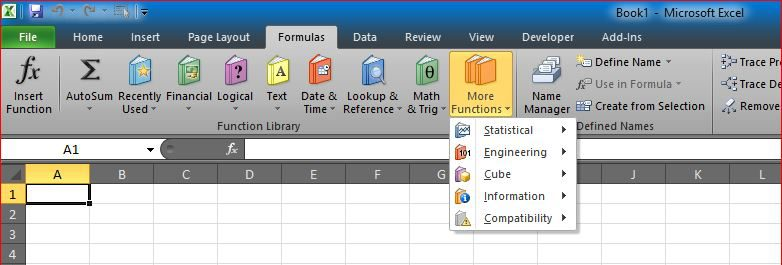# OFFSET+MATCH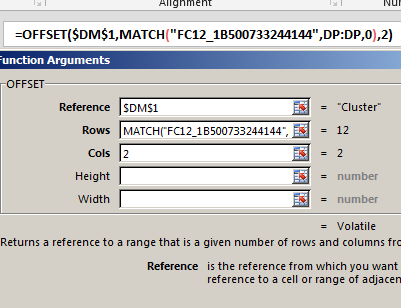One of the power of combining functions in one cell is using the best of these functions

An example is here, OFFSET + MATCH will allow you to do something similar to VLOOKUP.

### Why do we want to do that?

VLOOKUP will search in the left-most column in a table to get a value from a column on the right. But this is not the situation all the time

We needed several times to locate a row by using a column on the right, then get the value from a column left to it.

This is when VLOOKUP can not help us.

And this is when a combined function like OFFSET + MATCH can get the job done.

So, if we have a table as below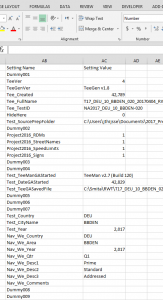A simple VLOOKUP can help us find any of these values, say “Test_Country”

`=VLOOKUP("Test_Country",AB:AC,2,FALSE)`

Which will return the expected result.

However, a table as below, we cannot use VLOOKUP if we want to search using “TreeKey” column, or “UnitID”, basically any column on the right of the table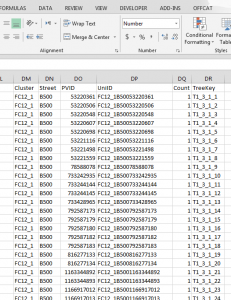Since VLOOKUP searches only left-most column of table.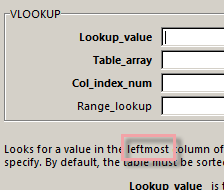And if we try using -ve number in “Col_index_num” we get an error

### So, what can we do?

Use combination of OFFSET and MATCH

MATCH will do the actual search in the needed column, while OFFSET gives me the actual return as below

below example will search for “T1_3_1_9” in column “TreeKey” column and returns the “PVID” of that item

`=OFFSET(\$DM\$1,MATCH("T1_3_1_9",DR:DR,0),2)`

Or, we can search for “FC12_1B500733244144” in “UnitID” column and return its “PVID” as below

`=OFFSET(\$DM\$1,MATCH("FC12_1B500733244144",DP:DP,0),2)`

Hope this will help